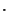Next: Experiments Up: Using Text Categorization Techniques Previous: Related Work

# Review of K-Nearest Neighbor Text Categorization Method

Text categorization is the process of grouping text documents into one or more predefined categories based on their content. A number of statistical classification and machine learning techniques have been applied to text categorization, including regression models, Bayesian classifiers, decision trees, nearest neighbor classifiers, neural networks, and support vector machines .

The first step in text categorization is to transform documents, which typically are strings of characters, into a representation suitable for the learning algorithm and the classification task. The most commonly used document representation is the so-called vector space model. In this model, each document is represented by a vector of words. A word-by-document matrix A is used for a collection of documents, where each entry represents the occurrence of a word in a document, i.e.,, whereis the weight of word i in document j. There are several ways of determining the weight. Letbe the frequency of word i in document j, N the number of documents in the collection, M the number of distinct words in the collection, andthe total number of times word i occurs in the whole collection. The simplest approach is Boolean weighting, which sets the weightto 1 if the word occurs in the document and 0 otherwise. Another simple approach uses the frequency of the word in the document, i.e.,. A more common weighting approach is the so-called tfidf (term frequency - inverse document frequency) weighting:(1)

A slight variation  of the tfidf weighting, which takes into account that documents may be of different lengths, is the following:(2)

For matrix A, the number of rows corresponds to the number of words M in the document collection. There could be hundreds of thousands of different words. In order to reduce the high dimensionality, stop-word (frequent word that carries no information) removal, word stemming (suffix removal) and additional dimensionality reduction techniques, feature selection or re-parameterization , are usually employed.

To classify a class-unknown document X, the k-Nearest Neighbor classifier algorithm ranks the document's neighbors among the training document vectors, and uses the class labels of the k most similar neighbors to predict the class of the new document. The classes of these neighbors are weighted using the similarity of each neighbor to X, where similarity is measured by Euclidean distance or the cosine value between two document vectors. The cosine similarity is defined as follows:(3)

where X is the test document, represented as a vector;is the jth training document;is a word shared by X and;is the weight of wordin X;is the weight of wordin document;is the norm of X, andis the norm of. A cutoff threshold is needed to assign the new document to a known class.

 Terms Text categorization Intrusion Detection N total number of documents total number of processes M total number of distinct words total number of distinct system callsnumber of times ith word occurs number of times ith system call was issuedfrequency of ith word in document j frequency of ith system call in process jjth training document jth training process X test document test process

The kNN classifier is based on the assumption that the classification of an instance is most similar to the classification of other instances that are nearby in the vector space. Compared to other text categorization methods such as Bayesian classifier, kNN does not rely on prior probabilities, and it is computationally efficient. The main computation is the sorting of training documents in order to find the k nearest neighbors for the test document.

We seek to draw an analogy between a text document and the sequence of all system calls issued by a process, i.e., program execution. The occurrences of system calls can be used to characterize program behavior and transform each process into a vector. Furthermore, it is assumed that processes belonging to the same class will cluster together in the vector space. Then it is straightforward to adapt text categorization techniques to modeling program behavior. Table 1 illustrates the similarity in some respects between text categorization and intrusion detection when applying the kNN classifier.

There are some advantages to applying text categorization methods to intrusion detection. First and foremost, the size of the system-call vocabulary is very limited. There are less than 100 distinct system calls in the DARPA BSM data, while a typical text categorization problem could have over 15000 unique words . Thus the dimension of the word-by-document matrix A is significantly reduced, and it is not necessary to apply any dimensionality reduction techniques. Second, we can consider intrusion detection as a binary categorization problem, which makes adapting text categorization methods very straightforward.Next: Experiments Up: Using Text Categorization Techniques Previous: Related Work
Yihua Liao 2002-05-13